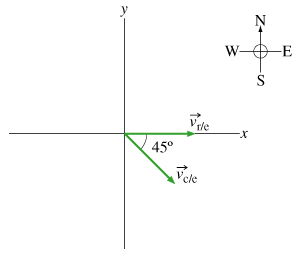# Problem: A canoe has a velocity of 0.400 m/s southeast relative to the earth. The canoe is on a river that is flowing at 0.460 m/s east relative to the earth. (Figure 1) Find the magnitude of the velocity vc/r of the canoe relative to the river.Express your answer in meters per second.

###### FREE Expert Solution

Vector magnitude,

$\overline{)\mathbf{|}\stackrel{\mathbf{⇀}}{\mathbf{v}}\mathbf{|}{\mathbf{=}}\sqrt{{{\mathbf{v}}_{\mathbf{x}}}^{\mathbf{2}}\mathbf{+}{{\mathbf{v}}_{\mathbf{y}}}^{\mathbf{2}}}}$

Resolving vector components,

Let positive y-axis point to the north and positive x-axis point to the east.

Resolving components,

${\stackrel{\mathbf{⇀}}{\mathbf{v}}}_{\mathbf{r}\mathbf{/}\mathbf{e}}$= 0.460 m/s (cos 0° i + sin 0° j) = 0.460 m/s i + 0 m/s j

${\stackrel{\mathbf{⇀}}{\mathbf{v}}}_{\mathbit{c}\mathbf{/}\mathbf{e}}$= 0.400 m/s {cos (- 45°) i + sin (- 45°) j } = 0.283 m/s i - 0.283 m/s j

89% (339 ratings)###### Problem Details

A canoe has a velocity of 0.400 m/s southeast relative to the earth. The canoe is on a river that is flowing at 0.460 m/s east relative to the earth. (Figure 1)Find the magnitude of the velocity vc/r of the canoe relative to the river.